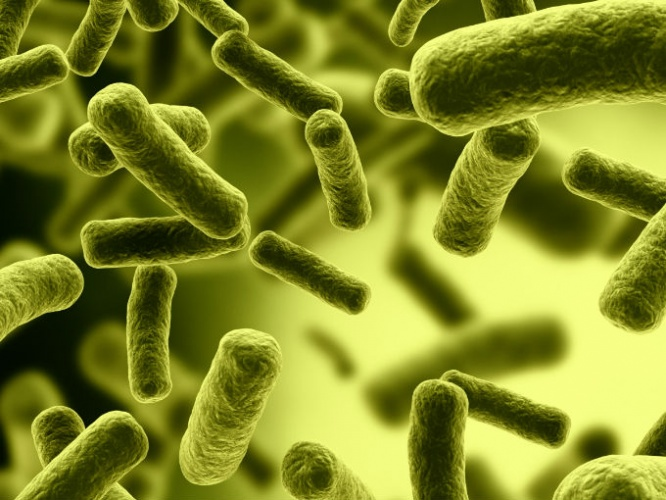# Exponential growth

Calculus Level 5In a certain biology experiment rate of increase of number of bacterias at time t is found to be numaricaly equal to number of bacterias prasent at that time,if a denotes the number of bacterias prasent initialy and b denotes number of bacterias prasent after time t(in seconds) then find out the ratio of b and a after 10s Now suppose your answer is n then find out the value of ⌊n⌋

×